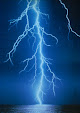## Sunday, January 29, 2012

Voltage Notching is described by IEEE as a recurring power quality disturbance due to the normal operation of power electronic devices (i.e. rectifier), when current is commutated from one phase to another. Conventionally, the current waveform is used as the starting point for harmonic analysis, and voltage notching is simply derived from the IZ drops of the harmonic currents.

In addition, voltage notching characterizes an unusual case that falls between harmonics and transients. This is because notching takes place in steady-state, thus, it can be distinguished by the harmonic spectrum of the affected voltage. On the other hand, the components of the frequency related to voltage notching are somewhat high and may not be promptly categorized with a measurement device commonly employed for harmonic analysis.Voltage Notching
Sources

Voltage Notching is primarily caused by three-phase rectifiers or converters that generate continuous DC current. As mentioned, the voltage notches happen when the current commutates from one phase to another. Subsequently, a momentary short circuit between two phases will occur during this period.

Moreover, the depth of the notch at any point in the system is influenced by the source inductance, the isolating inductance between the rectifier/converter, as well as the point being examined. Consequently, the width of the notch is the commutation angle.

µ = cos-1[cos α – (Xs+Xt)Idc] - α
cos µ = 1 – (2Ex/Edo)

where:
µ = commutation angle
α = delay angle
Xs = system reactance in per unit on converter base
Xt = converter transformer reactance in per unit on converter base
Idc = DC current in per unit on converter base
Ex = direct-voltage drop caused by commutating reactance
Ed0 = theoretical direct voltage

Effects and Solution

Voltage Notches introduce harmonic and non-harmonic frequencies that are much higher than those found in higher voltage systems. Usually, these frequencies are in the radio frequency range, that cause negative operational effects, such as signal interference introduced into logic and communication circuits. Also, when of sufficient power, the voltage notching effect may overload electromagnetic interference filters, and other similar high-frequency sensitive capacitive circuits.

Furthermore, the solution for voltage notching typically involves isolation of the critical and sensitive equipment from the source (i.e. rectifiers) of the power quality problem.

Synopsis:

Source: Electronic Devices (e.g. converters)
Symptoms: Malfunction
Occurrence: Very Low
Mitigation: Isolation

References:
IEEE 519-1992. Recommended Practice and Requirements for Harmonic Control in Electrical Power Systems
IEEE 1159-1995. Recommended Practice for Monitoring Electric Power Quality